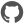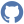# Methods

Namespace: global
Parent Module: ExpressionOptimizer

### Functions and values

 Function or value Description ``` ( cut not used condition ) e ``` Signature: e:Expression -> Expressionif false then x else y -> y CompiledName: `cut not used condition` ``` ( evaluate basic constant math ) e ``` Signature: e:Expression -> ExpressionEvaluate simple math between two constants. 9 * 3 --> 27 "G" + "G" --> "GG" CompiledName: `evaluate basic constant math` ``` ( evaluate constants ) e ``` Signature: e:Expression -> ExpressionEvaluating constants to not mess with our expressions: CompiledName: `evaluate constants` ``` ( not false is true ) e ``` Signature: e:Expression -> Expressionnot(false) -> true CompiledName: `not false is true` ``` ( remove AnonymousType ) e ``` Signature: e:Expression -> ExpressionPurpose of this is to replace non-used anonymous types: new AnonymousObject(Item1 = x, Item2 = "").Item1 --> x CompiledName: `remove AnonymousType` ``` ( replace constant comparison ) e ``` Signature: e:Expression -> ExpressionPurpose of this is optimize away already known constant=constant style expressions. 7 > 8 --> False "G" = "G" --> True CompiledName: `replace constant comparison` ``` absorb _arg1 ``` Signature: _arg1:Expression -> Expression``` annihilate _arg1 ``` Signature: _arg1:Expression -> Expression``` associate _arg1 ``` Signature: _arg1:Expression -> ExpressionNot in use, would cause looping... ``` balancetree _arg1 ``` Signature: _arg1:Expression -> ExpressionBalance tree that is too much weighted to other side. The real advantage is not-so-nested-stack ``` commute _arg1 ``` Signature: _arg1:Expression -> Expression``` complement _arg1 ``` Signature: _arg1:Expression -> Expression``` deMorgan _arg1 ``` Signature: _arg1:Expression -> Expression``` distribute _arg1 ``` Signature: _arg1:Expression -> ExpressionNot in use, would cause looping... ``` doubleNegation _arg1 ``` Signature: _arg1:Expression -> Expression``` gather _arg1 ``` Signature: _arg1:Expression -> Expression``` idempotence _arg1 ``` Signature: _arg1:Expression -> Expression``` identity _arg1 ``` Signature: _arg1:Expression -> Expression